Three resistances 2Ω, 4Ω, 5Ω are combined in series and this combination is connected to a battery of 12 V emf and negligible internal resistance. The potential drop across these resistances are
• a)
(5,45,4.36,2.18)V
• b)
(2,18,5.45,4.36)V
• c)
(4.36,2.18,5.45)V
• d)
(2.18,4.36,5.45)V

### Related TestBs Academy Feb 13, 2022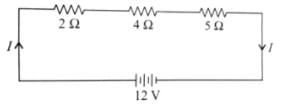Let current in the circuit is I. Then total resistance in the circuit.
R = R1 + R2 + R3 = 2 + 4 + 5 = 11Ω
∴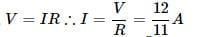The potential drop across 2Ω resistance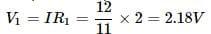The potential drop across 4Ω resistance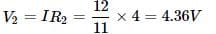The potential drop across 5Ω resistance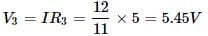Hence(V1, V2, V3) = (2.18, 4.36, 5.45)V

Let current in the circuit is I. Then total resistance in the circuit.R = R1 + R2 + R3 = 2 + 4 + 5 = 11Ω∴The potential drop across 2Ω resistanceThe potential drop across 4Ω resistanceThe potential drop across 5Ω resistanceHence(V1, V2, V3) = (2.18, 4.36, 5.45)V
Do you know? How Toppers prepare for NEET Exam
With help of the best NEET teachers & toppers, We have prepared a guide for student who are preparing for NEET : 15 Steps to clear NEET Exam